Electricity and Magnetism

# Kirchoff's Current Law (conservation at nodes)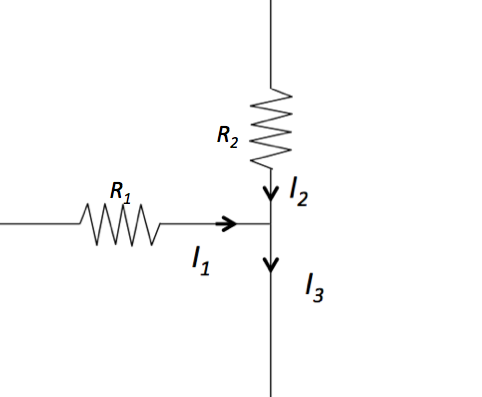The above figure shows part of a circuit. When the currents coming through $$R_1 = 6 \Omega$$ and $$R_2 = 4 \Omega$$ are $$I_1 = 8 \text{ A}$$ and $$I_2 = 2 \text{ A},$$ respectively, what is $$I_3?$$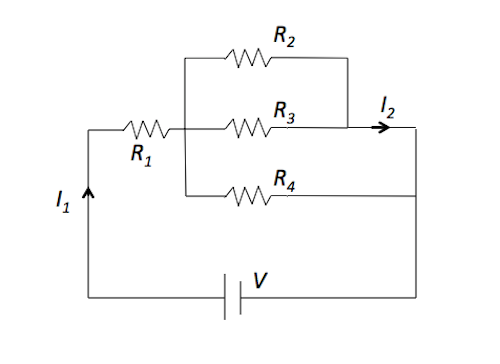In the above circuit, the resistance $$R_1$$ is $$8 \Omega$$ and the other resistances $$R_2, R_3,$$ and $$R_4$$ are $$4 \Omega$$ each. If $$I_1 = 21 \text{ A},$$ what is the current $$I_2?$$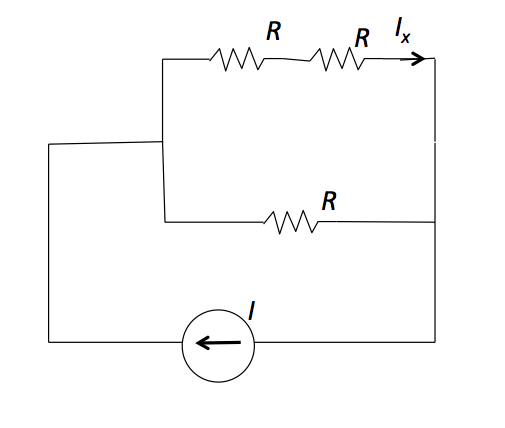In the above circuit, the current source flow is $$I = 21 \text{ A}.$$ When the resistances are given by $$R = 3 \Omega,$$ find the current $$I_x.$$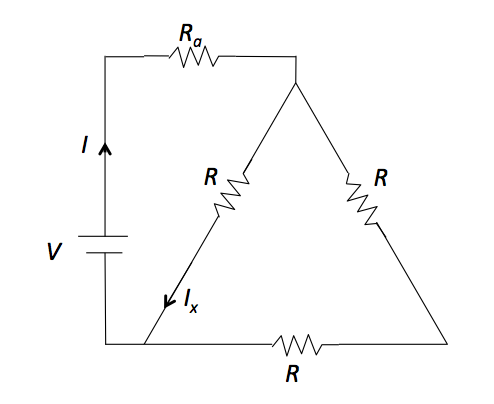In the above circuit, let the resistances $$R_a = 7 \Omega$$ and the resistances $$R = 2 \Omega.$$ The voltage of battery is unknown but the current is $$I = 18 \text{ A}.$$ Find the current $$I_x$$ in the above circuit.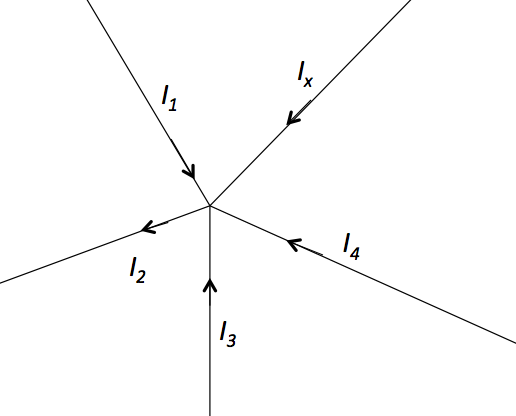In the above circuit, $$I_1 = 4 \text{ A},$$ $$I_2 = 8 \text{ A},$$ $$I_3 = 3 \text{ A}$$ and $$I_4 = 3 \text{ A}.$$ What is $$I_x?$$

×

Problem Loading...

Note Loading...

Set Loading...